Disclosure: This post may contain affiliate links, meaning when you click the links and make a purchase, we receive a commission.

# Solving equations in Excel (polynomial, cubic, quadratic, & linear)

Excel has many features that can perform different tasks. Besides performing different statistical, financial analyses we can solve equations in Excel. In this article, we will analyze a popular topic which is Solving Equations in Excel.

In this article, we will solve different kinds of equations like cubic, polynomial, linear, quadratic with different features of Excel.

## Solving Polynomial Equations in Excel

A polynomial equation/function can be quadratic, linear, quartic, cubic and so on. The Polynomial equations don’t contain a negative power of its variables. Different kind of polynomial equations example is given below.

1) Monomial: y=mx+c
2) Binomial: y=ax2+bx+c
3) Trinomial: y=ax3+bx2+cx+d

With the direct calculation method, we will also discuss other methods like Goal Seek, Array, and Solver in this article to solve different polynomial equations.

Read More: 3D Referencing & External Reference in Excel

## Solve Cubic Equation in Excel using Goal Seek

Let`s say we have a cubic equation which is Y=5x3-2x2+3x-6. We will solve this equation for finding the value of “X” with a specific value of “Y”. We will use the Excel Goal Seek feature here to solve the equation. The procedure is given below.

• First set the coefficients in different cells.
• Set the initial value of X as “0” in cell B6.
• After that write the polynomial equation in cell G3 with respect to the cells of coefficients and the initial value of X.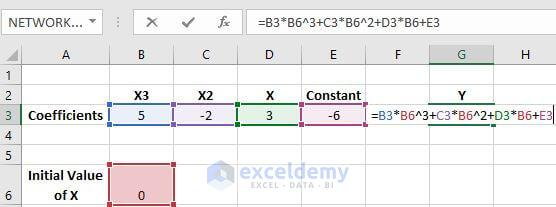• Now, under the Data tab click on to the Goal Seek option under the Forecast option.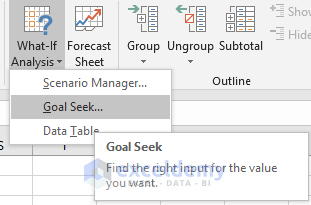• In the Goal Seek dialogue box, insert the inputs as follows and press on OK.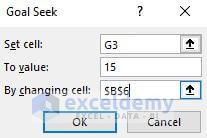• The Goal Seek feature will do some iterations for the final value of Y which is set as 15 in this example.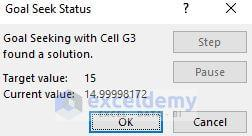• After the Goal Seek completes its operation you will see the initial value of X and the cell where the formula was placed is changed. Now the initial value is showing the value of X when the value of Y becomes 15.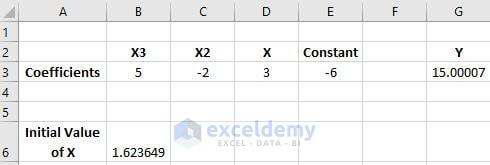## Solve Cubic Equation in Excel using Solver

You can also use the Solver feature of Excel to solve cubic equations. Let`s solve the previous equation for a better understanding.

• Just after typing the equation in cell G3, click on to solver which is under Analysis option of Data tab.
• In the Solver Parameters dialogue box, do the following and press the Solve option.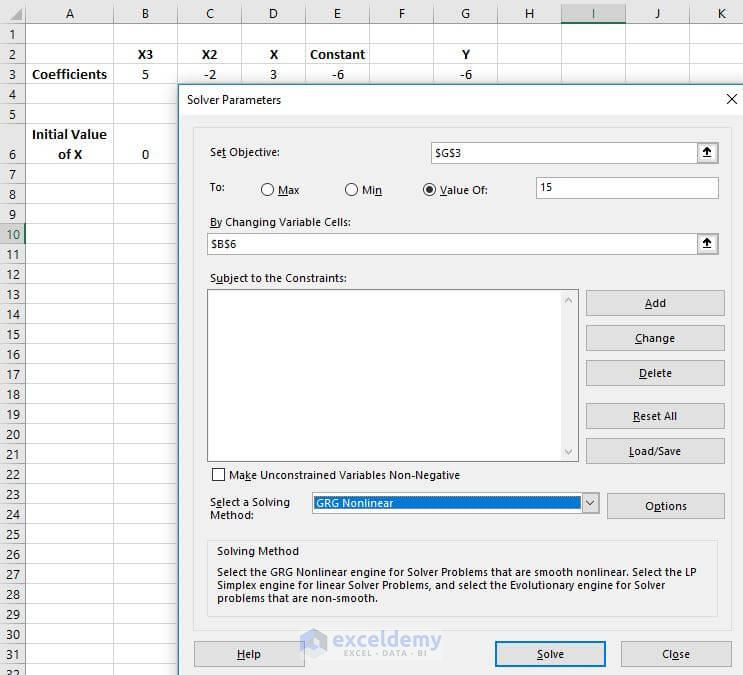• Here we set the target value 15. B6 is the variable cell here. As it is a non-linear equation the solving method was selected as GRG Nonlinear.
• The below result will be found after the operation.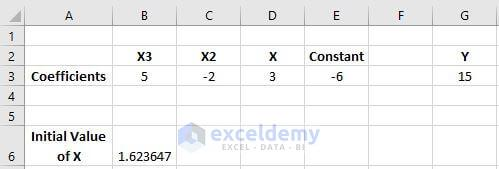Read More: How to use Goal Seek to find an input value

## Solve Quadratic Equation in Excel using Goal Seek Feature

Let`s solve a quadratic equation which is Y=3x2+6x-5. We will see how this equation can be solved with the Goal Seek feature. The procedures are given below.

• First set the coefficients in different cells.
• Set the initial value of X as “0” in cell B6.
• After that write the equation in cell F3 with respect to the cells of coefficients and the initial value of X.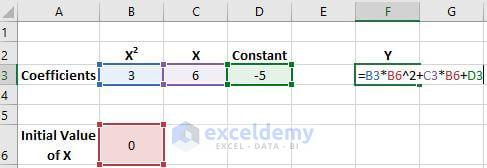• Now, under the Data tab click on to the Goal Seek option under the Forecast option.• In the Goal Seek dialogue box, insert the inputs as follows and press on OK.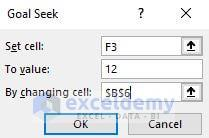• The Goal Seek feature will do some irritations for the final value of Y which is set as 12 in this example.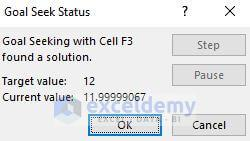• After the Goal Seek completes its operation you will see the initial value of X and the cell where the formula was placed is changed. Now the initial value is showing the value of X when the value of Y becomes 12.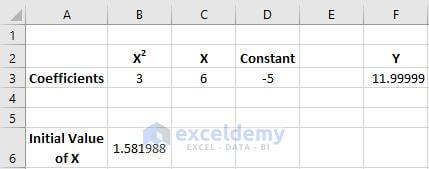Read More: Data Analysis in Excel – Learn 10 Common Statistical Terms

## Use of Solver to Solve Quadratic Equation in Excel

You can also use the Solver feature of Excel to solve quadratic equations. Let`s solve the same equation for a better understanding.

• Just after typing the equation in cell F3, click on to solver which is under Analysis option of Data tab.
• In the Solver parameters dialogue box, do the following and press Solve.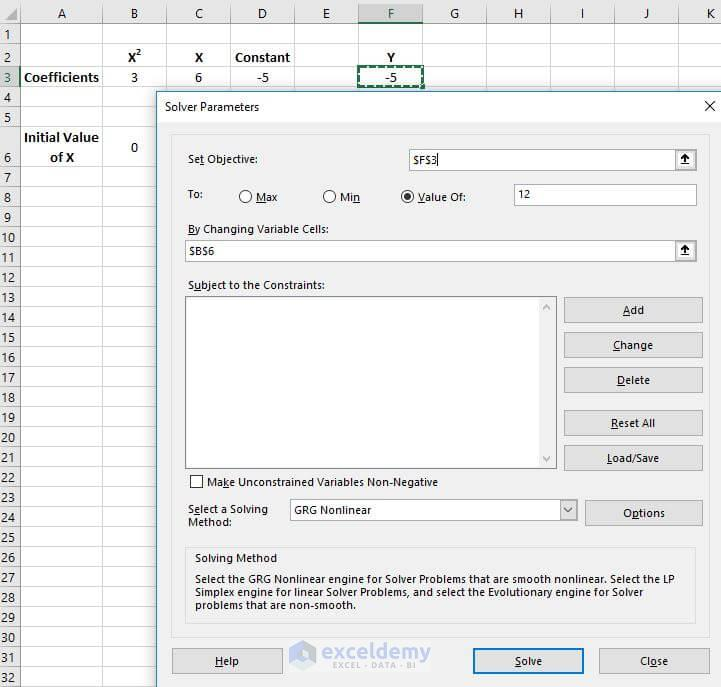• Here we set the target value 15. B6 is the variable cell here. As it is a non-linear equation the solving method was selected as GRG Nonlinear.
• The below result will be found after the operation.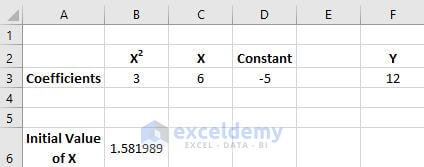## Solve Quadratic Equation in Excel using Formula

The format of a quadratic equation is x=(-b±√(b^2-4ac))/2a .By using this formula directly we can find the roots of the quadratic function. In the below picture we calculate the roots of the quadratic functions. Here the roots are X1 and X2.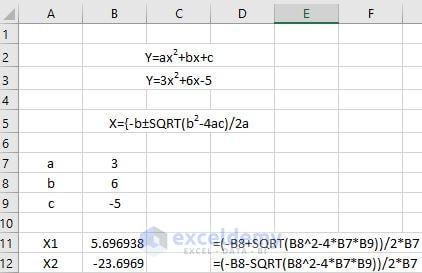## Solve Linear Equations in Excel with Matrix System

We can use matrix system to solve a set of linear equations in excel. Let`s say we have 3 equations where the values of x, y, and z are unknown. The equations are,

3x+2y+z=8……………… (1)
11x-9y+23=27…………..(2)
8x-5y=10….………………(3)

We will use the formula X=A-1B. Where both A-1 and B are the arrays. The procedure of solving the set of linear equations is given below.

• Write down the coefficients of x, y, and z along with constants in an array form just like the picture below. The coefficients of x, y and z are set of matrix A and the constants are set of matrix B.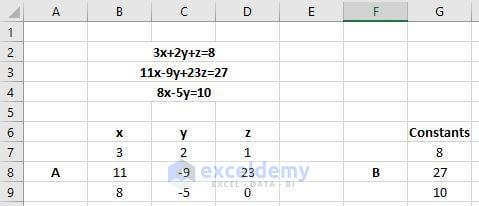• Now select the range B11:D13 and write down the formula =MINVERSE(B7:D9) and press Ctrl+Shift+Enter together to use this formula as an array.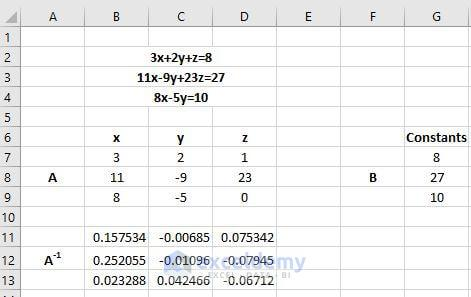• Now to find the result of X select the range G11:G13 and enter the formula =MMULT(B11:D13,G7:G9) and press Ctrl+Shift+Enter together to use this formula as an array. This function returns the matrix product of the two arrays. Here B11:D13 is the array set of A-1 and G7:G9 is the array set of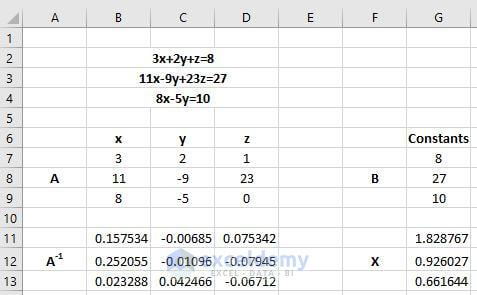## Solving Linear Equations in Excel Using Solver

It`s quite easy to find the variables of different linear equations using solver. The procedure is shown below.

• Make a table named Value of Variables in your worksheet and keep some blank spaces beside x, y, and z.
• Make another table which contains the equations with variables and the constants.
• In the Equations column (Column E) write down the equations, =3*C7+2*C8+C9, =11*C7-9*C8+23*C9 and =8*C7-5*C8. We use the formula =FORMULATEXT() to show these equations in column F. The constants are written in column G.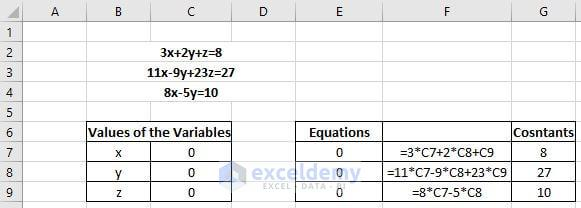• Click on to Solver which is under Analysis option of Data
• In Solver Parameters dialogue box, set the Set Objectives as \$E\$7, select the Value of option and type 8. In the by changing variable cells option write \$C\$7:\$C\$9. Uncheck the Make Unconstrained Variables Non-Negative and select Simplex LP in the Select a Solving Method Option.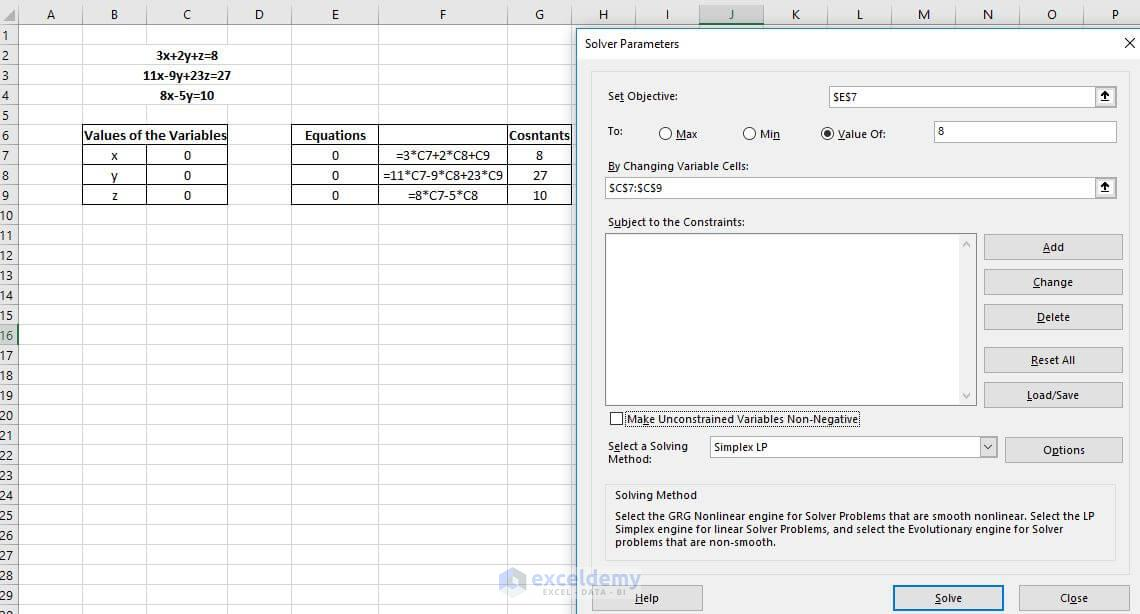Click to see the full image

• Press on to Add in the Solve Parameters dialogue box.
• In the Add Constraint, dialogue box, do the followings and press on to Add.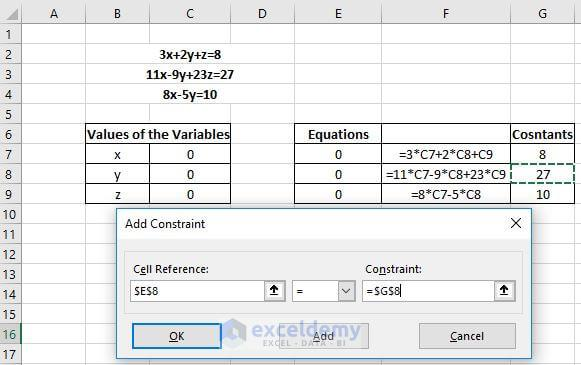• It`s time to gather the information of last equation as the input of solver. Do the followings and press OK.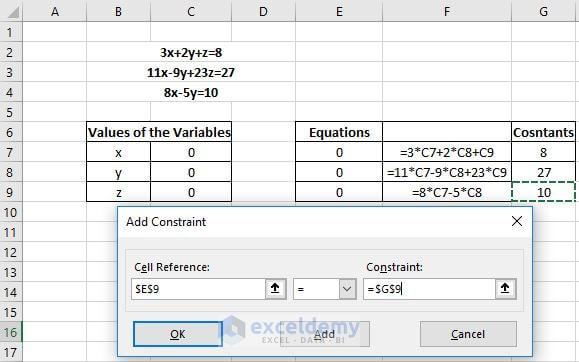• After this, your Solver Parameters will look like the picture below. Press on to the Solve option to continue the operation.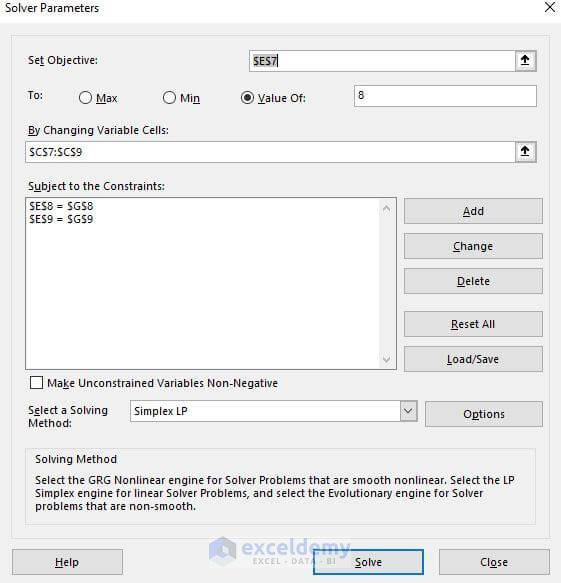• After you press on to the Solve option you will see the change in the Values of the Variables The values that you get in this table are the actual values of the variables x, y, and z.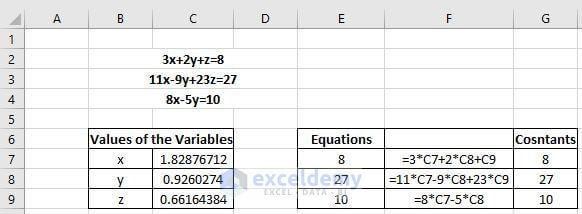Note: After you press on to the Solve option you will get a new dialogue box of Solver Results. Press on to the Keep Solver Solution and click OK to make the changes in your worksheet.

Related: How to solve simultaneous linear equations in Excel using Solver

## Conclusion

In this article, we see various processes of solving many different kinds of equations. Out of the many processes, the Solver in Excel is the best option. In Solver you can not only find the roots of the equation, you can also find the values of different variables simultaneously. The Solver in Excel makes it easy for the users to perform the linear programming problems.

Hello!

Welcome to my Profile. Here I will be posting articles related to Microsoft Excel. I have completed my BSc in Electrical and Electronic Engineering from American International University-Bangladesh. I am a diligent, goal-oriented engineer with an immense thirst for knowledge and attitude to grow continuously. Continuous improvement and life-long learning is my motto.

1. ReplyHoshi Tata May 8, 2018 at 9:37 AM

• ReplyMay 8, 2018 at 1:32 PM

Thanks for asking. It was creating more indexed pages in Google that was bad for us. What you can do is: just you can copy the whole article and paste into a word document and then convert it into a PDF file. There is little chance of getting back of that button.
Best regards

2. ReplyShawn S Fahrer Nov 9, 2019 at 7:57 AM

I have a few points that when graphed LOOK like a quadratic equation. How do I go from the set of points to get EXCEL to deliver an EQUATION for the quadratic function given those points (like it can do for LINEAR equations)?

3. ReplyManuj Dec 20, 2019 at 5:55 AM

Hi thanks for posting this article. Can you please elaborate on why did you assume 15 as the value of Y? By definition, shouldn’t we be solving for 3 values of x as the equation is cubic?